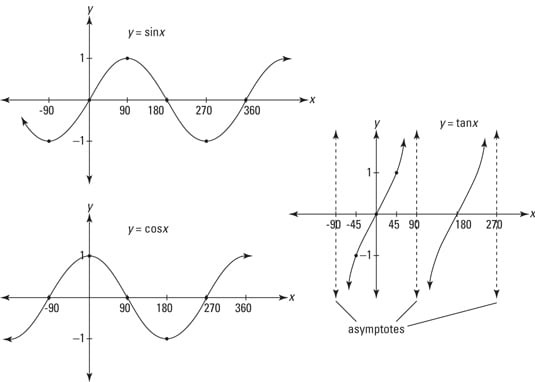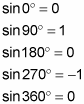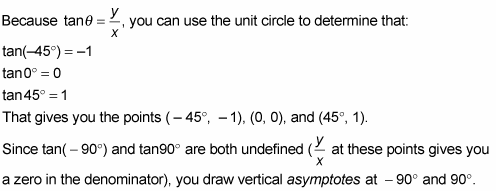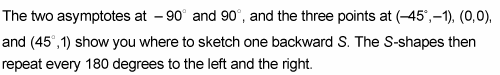##### Differential Equations For DummiesSo, you need to graph a sine, cosine, or tangent function. Sine, cosine, and tangent — and their reciprocals, cosecant, secant, and cotangent — are periodic functions, which means that their graphs contain a basic shape that repeats over and over indefinitely to the left and the right. The period of such a function is the length of one of its cycles.If you know the unit circle, you can easily reproduce these three graphs by hand. First, note that the sine and cosine graphs are the same shape — cosine is the same as sine, just slid 90 degrees to the left. Also, notice that their simple wave shape goes as high as 1 and as low as –1, and goes on forever to the left and right, repeating every 360 degrees. That’s the period of both functions, 360 degrees. (It’s no coincidence, by the way, that 360 degrees is also once around the unit circle.)

The unit circle tells you thatIf you start with these five points, you can sketch one cycle. The cycle then repeats to the left and right. You can use the unit circle in the same manner to sketch the cosine function.

In the preceding figure, you can see that the period of the tangent function is 180 degrees. If you remember that and the basic pattern of repeating backward S-shapes, sketching it isn’t difficult.An asymptote is an imaginary line that a curve gets closer and closer to but never touches.